## Modeling Efficient Multiplication and Division Operations for FPGA Targeting

These guidelines illustrate the recommended settings when using Divide and Product blocks in your model for improved area and timing on the target FPGA. Each guideline has a severity level that indicates the level of compliance requirements. To learn more, see HDL Modeling Guidelines Severity Levels.

### Designing Multipliers and Adders for Efficient Mapping to DSP Blocks on FPGA

Guideline ID

`2.7.1`

Severity

Strongly Recommended

Description

Digital signal processing (DSP) algorithms use several multipliers and accumulators. FPGA devices provided by vendors such as Xilinx® and Intel® contain dedicated DSP slices. These small size, high speed, DSP slices contain several multipliers and accumulators that make FPGA devices best suited for DSP applications.

The architecture of DSP slices varies widely across the different FPGA vendors and across different families of devices provided by the same vendor. To map your Simulink® model containing adders, multipliers, and delays to DSP slices, adapt your model to the DSP slice architecture by taking into consideration:

• Arrangement of flipflops, adders, and multipliers in the DSP slice.

• Rounding and saturation settings.

• Bit widths of the adders and multipliers. For efficient mapping, use bit widths in your model that are less than or equal to the bit widths of the DSP unit.

When the bit widths in your model become larger than the bit widths of the DSP, your design does not fit onto one DSP. In this case, multiple DSPs or additional logic is required.

You can map these blocks in your model to DSP blocks on an FPGA:

• Delay

• Product

• Multiply-Accumulate

This figure illustrates the Xilinx DSP architecture. Xilinx 7 series FPGAs have dedicated DSP slices that use this architecture. The DSP architecture consists of input registers, pre-adder, 25x18 multiplier, intermediate registers, post-adder, and an output register.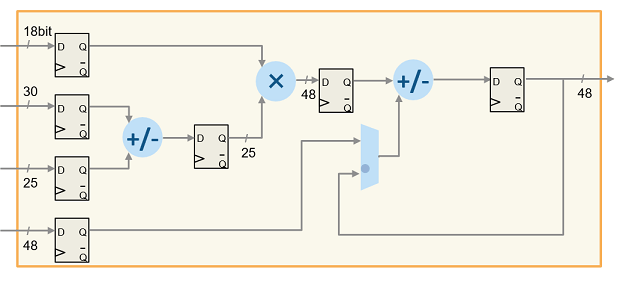This figure illustrates the Intel DSP architecture. This DSP architecture for Stratix® V devices is a variable precision DSP architecture. The DSP blocks can have bit widths of 9, 18, 27, and 36 bits, and 18x25 complex multiplication for FFTs.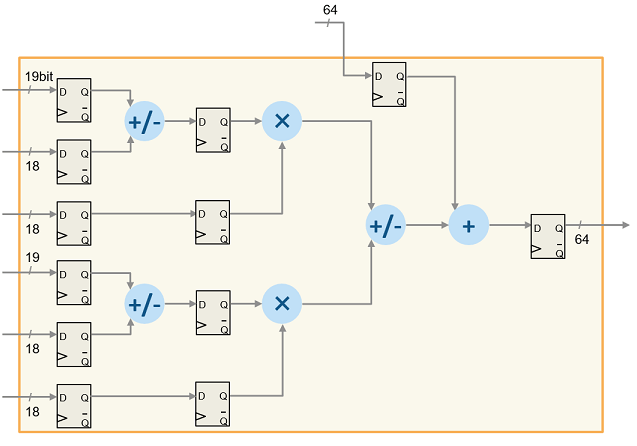To learn how you can design your algorithm to map to this DSP unit, open the model `hdlcoder_multiplier_adder_dsp.slx`

```open_system('hdlcoder_multiplier_adder_dsp') set_param('hdlcoder_multiplier_adder_dsp', 'SimulationCommand', 'Update') ```The model consists of two subsystems dsp_subsys1 and dsp_subsys2 that implement the operation C+((A+D)*B. You can also implement this operation by using Multiply-Add or Multiply-Accumulate blocks as illustrated by subsystems DSP_MultAdd and DSP_MultAcc.

`dsp_subsys1` implements the operation C+((A+D)*B by using bit widths that equal the DSP on a Xilinx 7 series FPGA. If you open the HDL Workflow Advisor and deploy this Subsystem onto a Xilinx Virtex® 7 FPGA, the entire design fits exactly onto one DSP slice.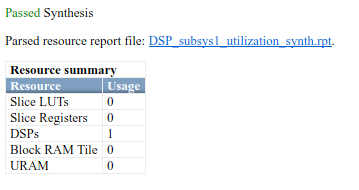`dsp_subsys2` implements the same operation by using bit widths that are larger than the DSP on a Xilinx FPGA. If you deploy this Subsystem onto a Xilinx Virtex 7 FPGA, you see that the entire design fits onto one DSP slice and uses additional slice logic.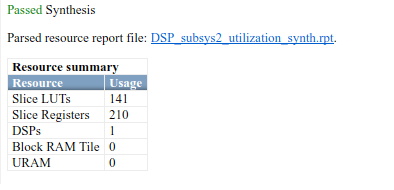### Set ConstMultiplierOptimization HDL Block Property to `auto` for Gain Block

`2.7.2`

#### Severity

Strongly Recommended

#### Description

When you use a Gain block in your design, to achieve the most area-efficient implementation, set the ConstMultiplierOptimization HDL block property to `auto`. The code generator chooses between `CSD` and `FCSD` implementations that yields the smallest circuit size and generates HDL code without using the multiplication (*) operator.

You can use this setting to avoid targeting DSP resources and reduce the number of logic circuits on Intel® Quartus® Prime when synthesizing your design on the target FPGA. For example, this table shows the generated HDL code for the Gain block depending on the HDL block property settings for the ConstMultiplierOptimization block.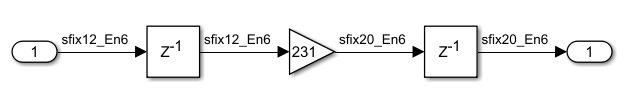ConstMultiplierOptimization Settings and Impact on Generated HDL Code

`ConstMultiplierOptimization` SettingOperationsGenerated HDL Code
`CSD`

Casts input data in parallel and adds or subtract the results.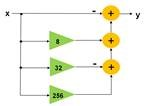This code shows the generated VHDL code.

```-- CSD Encoding(231): 1001'01001'; Cost (Adders) = 3 DOUT_mul_temp <= ((resize(DIN & '0' & '0' & '0' & '0' & '0' & '0' & '0' & '0', 21) - resize(DIN & '0' & '0' & '0' & '0' & '0', 21)) + resize(DIN & '0' & '0' & '0', 21)) - resize(DIN, 21); DOUT <= DOUT_mul_temp(19 DOWNTO 0); ```

This code shows the generated Verilog code.

```// CSD Encoding (231) : 1001'01001'; Cost (Adders) = 3 assign Gain_1 = {DIN, {DIN, 8'b00000000}}; assign Gain_2 = {{4{DIN}}, {DIN, 5'b00000}}; assign Gain_3 = {{6{DIN}}, {DIN, 3'b000}}; assign Gain_4 = {{9{DIN}}, DIN}; assign DOUT_mul_temp = ((Gain_1 - Gain_2) + Gain_3) - Gain_4; assign DOUT = DOUT_mul_temp[19:0]; ```
`FCSD`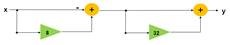This code shows the generated VHDL code.

```-- FCSD for 231 = 33 X 7; Total Cost = 2 -- CSD Encoding (33) : 0100001; Cost (Adders) = 1 Gain_factor <= resize(DIN & '0' & '0' & '0' & '0' & '0', 21) + resize(DIN, 21); -- CSD Encoding (7) : 1001'; Cost (Adders) = 1 DOUT_mul_temp <= resize(Gain_factor & '0' & '0' & '0', 21) - Gain_factor; DOUT <= DOUT_mul_temp(19 DOWNTO 0); ```

This code shows the generated Verilog code.

```// FCSD for 231 = 33 X 7; Total Cost = 2 // CSD Encoding (33) : 0100001; Cost (Adders) = 1 assign Gain_3 = {{4{DIN}}, {DIN, 5'b00000}}; assign Gain_4 = {{9{DIN}}, DIN}; assign Gain_factor = Gain_3 + Gain_4; // CSD Encoding (7) : 1001'; Cost (Adders) = 1 assign Gain_1 = {Gain_factor, 3'b000}; assign Gain_2 = Gain_1[20:0]; assign DOUT_mul_temp = Gain_2 - Gain_factor; assign DOUT = DOUT_mul_temp[19:0]; ```
`auto`Selects CSD or FCSD implementation that uses fewer adders.Generated HDL code is same as CSD or FCSD implementation.
`none`Uses multiplication operator (*).

This code shows the generated VHDL code.

```DOUT_mul_temp <= to_signed(2#011100111#, 9) * DIN; DOUT <= DOUT_mul_temp(19 DOWNTO 0); ```

This code shows the generated Verilog code.

```assign DOUT_mul_temp = 231 * DIN; assign DOUT = DOUT_mul_temp[19:0]; ```

### Use `ShiftAdd` Architecture of Divide Block for Fixed-Point Types

`2.7.3`

Recommended

#### Description

When you use fixed-point data types as inputs to the Divide block, specify the HDL Architecture of the block as `ShiftAdd` and then set the HDL block property UsePipelines to `on`. In this architecture, the block computes the result by using multiple shift and add operations. The operations are pipelined to achieve higher clock frequencies on the target FPGA device.

When you use floating-point data types as inputs to the Divide block, leave the HDL Architecture to default value of `Linear` and set the Floating Point IP Library to `Native Floating Point`.